# Methods and formulas for 2-Sample t

Select the method or formula of your choice.

## Confidence interval (CI)

### Formula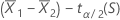to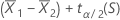### Notation

TermDescription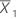mean of the first sample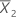mean of the second sample
tα/2 inverse cumulative probability of a t distribution at 1 – α/2
α 1 - confidence level / 100
s sample standard deviation as calculated for the test statistic

## T-value

### Formula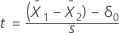The sample standard deviation, s, of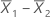depends upon the variance assumption.
Unequal variances

When you assume unequal variances, the sample standard deviation ofis:

The degrees of freedom are:

If necessary, Minitab truncates the degrees of freedom to an integer, which is a more conservative approach than rounding.

Equal variances
When you assume equal variances, the common variance is estimated by the pooled variance:
The standard deviation ofis estimated by:

The test statistic degrees of freedom are:

DF = n1 + n2 – 2

### Notation

TermDescriptionmean of the first samplemean of the second sample
ssample standard deviation ofδ0hypothesized difference between the two population means
s1sample standard deviation of the first sample
s2sample standard deviation of the second sample
n1sample size of the first sample
n2sample size of the second sample
VAR1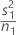VAR2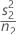## Calculate the pooled standard deviation

Suppose C1 contains the response, and C3 contains the mean for each factor level. For example:

C1 C2 C3
Response Factor Mean
18.95 1 14.5033
12.62 1 14.5033
11.94 1 14.5033
14.42 2 10.5567
10.06 2 10.5567
7.19 2 10.5567

1. Choose Calc > Calculator.
2. In Store result in variable, enter C4.
3. In Expression, enter SQRT((SUM((C1 - C3)**2)) / (total number of observations - number of groups)) . For the previous example, the Expression for the pooled standard deviation would be: SQRT((SUM(('Response' - 'Mean')**2)) / (6 - 2))

The value that Minitab stores is 3.75489.

## P-value

### Formula

The calculation for the p-value depends on the alternative hypothesis.

Alternative Hypothesis P-value
The degrees of freedom, DF, depend upon the variance assumption.
Unequal variances

When you assume unequal variances, the degrees of freedom are:

If necessary, Minitab truncates the degrees of freedom to an integer, which is a more conservative approach than rounding.

Equal variances

When you assume equal variances, the test statistic degrees of freedom are:

DF = n1 + n2 – 2

### Notation

TermDescription
μ1population mean of the first sample
μ1population mean of the second sample
n1 sample size of the first sample
n2 sample size of the second sample
δ0 hypothesized difference between the two population means
tt-statistic from the sample data
ta random variable from the t-distribution with DF degrees of freedom.
VAR1VAR2By using this site you agree to the use of cookies for analytics and personalized content.  Read our policy